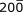## 2. Zeros

Most of the confusion with sig fig centers on zeros (0). There are times when a 0 is significant, and other times when it is merely a placeholder.  For example, do the following two distances mean the same thing?

200.00 ft

200 ft

Both express the same magnitude, but they differ in resolution. 200.00 implies a resolution to 0.01 (good to the nearest hundredth). How about 200? Is its resolution 1, 10, or 100?

The rules for zeros are:

(1) Zeros between nonzero digits are significant:  203   30501

(2) Zeroes to the left of a measurement are not significant:  00
5  00.0025

(3) Zeroes to the right of a measurement are only significant if a decimal point also appears in the quantity:  82.0  15.3600

Although these cover most situations, there can still be confusion when encountering zeros. How many sig fig does 200 have? According to the rules, both zeros would be merely place holders indicating magnitude but not accuracy, so 200 would have 1 sig fig.

But what if both zeros are significant (e.g., distance measure to the nearest foot)? How do we write 200 to show 3 sig fig?

One way would be to include a bar ( ¯ ) over the last significant 0:The disadvantage to this is that most people interpret a bar over a number as an infinitely repeating digit. It’s also sometimes hard to see. On top of that, how do you enter that on a computer keyboard?

Another would be to place a decimal point after the second 0:  200.

Unfortunately the decimal point often gets “lost” when people look at the number. Or more often, we just leave it off.

The best way is to express the number in scientific notation:    2.00x102

2.00 is the coefficient and is the number subject to error. This uses the last rule of zeros - zeros to the right of the decimal point are significant.

102 is the base and is an exact multiplier (100 exactly).

What if the first zero (the one immediately after the 2) is significant and the second is a place holder? How would you record it showing 2 sig fig?

A bar over the first 0 making it significant, or,

2.0x102 using scientific notation.